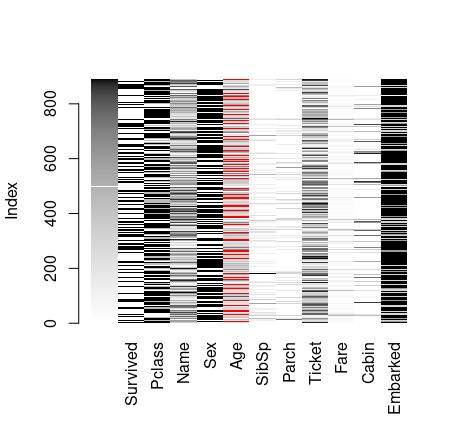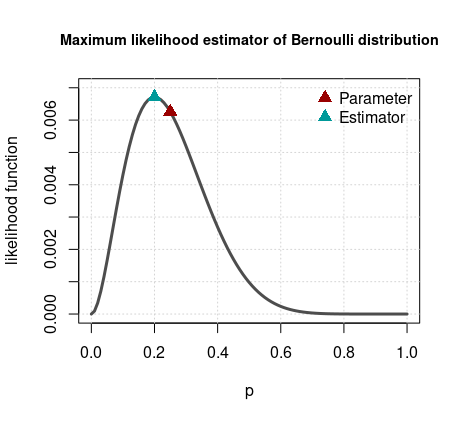# Logistic regression

The aim of this tutorial is to show how to write your own program for estimating a logistic regression. Note that the goal is purely educational since the logistic regression has already solid implementations in R.

### Introduction

Logistic regression is one of most famous tool to model binary data. It is often introduced in under-graduate class of statistics and machine learning.

For illustration, we fit a logistic regression on the titanic dataset. This dataset is made available for example via the titanic R package. You can obtain more detail on this dataset by calling the help ? titanic_train, read the package documentation or the Kaggle competition webpage. When installed, R comes already equipped with a rich library called stats which contains the glm function that allows to fit Generalized Linear Model, a broad class of model that includes logistic regression. In the next lines of code, we see an example using this glm function on the titanic dataset.

## decomment if not installed
## install.packages("titanic")

library(titanic) # call the library
data("titanic_train") # load the training dataset

fit_logistic <- glm(Survived ~ Pclass + Sex + Age + SibSp +
Parch + Fare,
data = titanic_train)
summary(fit_logistic)

1. We try to explain the binary data Survived with six different covariates, or explanatory variables.
2. The glm function takes as first argument a formula object, here Survived ~ Pclass + Sex + Age + SibSp + Parch + Fare (check ? formula for more details).
3. The family argument of glm specifies the distribution; for logistic regression it is specified to binomial(link = "logit"). 4. The data argument gives the dataset so names can be directly used in the formula.

The output of the summary is

Call:
glm(formula = Survived ~ Pclass + Sex + Age + SibSp + Parch +
Fare, family = binomial(link = "logit"), data = titanic_train)

Deviance Residuals:
Min       1Q   Median       3Q      Max
-2.7953  -0.6476  -0.3847   0.6271   2.4433

Coefficients:
Estimate Std. Error z value Pr(>|z|)
(Intercept)  5.389003   0.603734   8.926  < 2e-16 ***
Pclass      -1.242249   0.163191  -7.612 2.69e-14 ***
Sexmale     -2.634845   0.219609 -11.998  < 2e-16 ***
Age         -0.043953   0.008179  -5.374 7.70e-08 ***
SibSp       -0.375755   0.127361  -2.950  0.00317 **
Parch       -0.061937   0.122925  -0.504  0.61436
Fare         0.002160   0.002493   0.866  0.38627
---
Signif. codes:  0 ‘***’ 0.001 ‘**’ 0.01 ‘*’ 0.05 ‘.’ 0.1 ‘ ’ 1

(Dispersion parameter for binomial family taken to be 1)

Null deviance: 964.52  on 713  degrees of freedom
Residual deviance: 635.81  on 707  degrees of freedom
(177 observations deleted due to missingness)
AIC: 649.81

Number of Fisher Scoring iterations: 5

The column Estimate returns the estimators of the logistic regression; this is the quantities we are going to compute in this tutorial. Note that there are 177 observations missing.

We can for example visualize these missing values with the VIM library.

## decomment if not installed
## install.packages("VIM")
library(VIM)
matrixplot(titanic_train)It appears clearly that the variable Age is the only one missing values. There are different way to deal with missing values (glm simply delete the corresponding rows). Here for simplicity we will not consider Age so we have the following model:

fit_logistic2 <- glm(Survived ~ Pclass + Sex + SibSp +
Parch + Fare,
data = titanic_train)
summary(fit_logistic2)
Call:
glm(formula = Survived ~ Pclass + Sex + SibSp + Parch + Fare,
family = binomial(link = "logit"), data = titanic_train)

Deviance Residuals:
Min       1Q   Median       3Q      Max
-2.2340  -0.6786  -0.4817   0.6315   2.5515

Coefficients:
Estimate Std. Error z value Pr(>|z|)
(Intercept)  3.147350   0.375154   8.389  < 2e-16 ***
Pclass      -0.835995   0.126848  -6.591 4.38e-11 ***
Sexmale     -2.759428   0.195930 -14.084  < 2e-16 ***
SibSp       -0.256350   0.100785  -2.544    0.011 *
Parch       -0.088766   0.113191  -0.784    0.433
Fare         0.003416   0.002355   1.451    0.147
---
Signif. codes:  0 ‘***’ 0.001 ‘**’ 0.01 ‘*’ 0.05 ‘.’ 0.1 ‘ ’ 1

(Dispersion parameter for binomial family taken to be 1)

Null deviance: 1186.66  on 890  degrees of freedom
Residual deviance:  816.75  on 885  degrees of freedom
AIC: 828.75

Number of Fisher Scoring iterations: 5

### Logistic regression model

In probability theory, a random variable $Y$ that takes value $1$ with probability $p$ and $0$ with probability $1-p$ is called a Bernoulli random variable. We can write this statement as $\Pr(Y=1)=p$ and $\Pr(Y=0)=1-p$ where $p\in(0,1)$ is often called the probability of success. We can write the same statement more compactly as $$\Pr(Y)=p^Y(1-p)^{1-Y}.$$

The logistic regression model replaces this probability of success by the sigmoid function $$\sigma(t)=\frac{1}{1+e^{-t}}.$$ Generally we express further $t$ as a linear combination of known covariates $x$ and unknown coefficients $w$ as follows: $$t = w _0 + \sum _{j=1} ^{p} x _j w _j,$$

or more compactly in matrix form
$$t = Xw,$$

where the first column of $X$ is $1$’s and $w=(w _0, \ldots , w _{p})^{T}$. In this tutorial we are interested in estimating the coefficients $w$ and we are going to use the maximum likelihood estimator (MLE).

### MLE for Bernoulli random variables

Maximum likelihood estimator? Suppose you obtained $n$ observations, for example as follows:

p <- .25
n <- 10
set.seed(9842)
y <- rbinom(n,size=1,prob=p)
y

(note that we use rbinom() as Bernoulli distribution is a special case of the binomial distribution, which maybe also explain the family argument used before.)

  0 0 0 0 0 0 0 1 0 1

but you don’t know the parameter $p$. So a question is, what is this value? One way to answer this problem is to consider the likelihood function, a function that measures how probable is a given data as a function of the parameter. Denote this function by $\mathcal{L}(p;y)$. The idea is to find the value $p$ that maximizes $\mathcal{L}(p;y)$. For example, if we have two values $p_1,p_2$ and we have $\mathcal{L}(p_1;y)>\mathcal{L}(p_2;y)$, then $p_1$ is more plausible than $p_2$, there is more chance the data was generated by $p_1$ than by $p_2$. The maximum of this likelihood function is called the maximum likelihood estimator.

For $n$ i.i.d. Bernoulli random variables, the likelihood function is

$$\mathcal{L}(p;y)=\prod_{i=1}^n p^{y_i}\left(1-p\right)^{1-y_i}$$

or equivalently $$p^{s_n}\left(1-p\right)^{n-s_n},$$ where $s _n = \sum _{i=1} ^n y _i .$

We illustrate this function below.

## define the likelihood function
f <- function(p,y){
p^sum(y) * (1-p)^(length(y) - sum(y))
}
p_hat <- mean(y) # maximum likelihood estimator

## plot the likelihood function
plot(NA, xlim = c(0,1), ylim=c(0,7e-3),
xlab = "p", ylab = "likelihood function",
main = "Maximum likelihood estimator of Bernoulli distribution",
cex.main = .9)
grid()
curve(f(p,y),from = 0,to = 1, xname = "p",
lwd = 3, add = TRUE, col = "gray30")
points(x = p, y = f(p,y), pch = 17, col = "#990000", cex = 1.5)
points(x = p_hat, y = f(p_hat,y), pch = 17, col = "#009999", cex = 1.5)
legend("topright", legend = c("Parameter","Estimator"),
col = c("#990000","#009999"), pch = 17, pt.cex = 1.5,
bty = "n")We clearly see the likelihood function is a concave function (how would you verify this analytically?), so one way to compute its maximum is to find the point such that the slope of the function is zero. It is generally more convenient to work with the log-likelihood function $\ell(p;y)=\log(\mathcal{L}(p,y))$. Taking the logarithm does not change the maximum. The first derivative of the log-likelihood is

$$\frac{ \partial \ell(p;y) }{ \partial p} = \frac{s _n}{p} - \frac{ n - s _n}{1-p}.$$

Solving $\partial\ell(p;y)/\partial p = 0$ leads to the unique solution $\hat{p} = s _n / n$ (if $0 < s _n / n < 1$).

### MLE for logistic regression

For the logistic regression, the computations are slightly more involved. Suppose you observe $n$ binary data, the likelihood function is $$\mathcal{L}(w;y,X)=\prod_{i=1}^n\sigma(t_i)^{y_i}\left(1-\sigma(t_i)\right)^{1-y_i}.$$ Similarly, le log-likelhood is given by

$$\ell(w;y,X) = \sum _{i=1} ^{n} y _{i} \log\left(\frac{\sigma(t _{i})}{ 1 - \sigma(t _{i})}\right) + \log\left(1-\sigma(t _{i})\right),$$

where $$\sigma(t _{i}) = \frac{1}{ 1 + \exp( -x _{i}w)}$$

and $x _i$ is the ith row of $X$ (row-vector). Some manipulations lead to a simpler expression: $$\ell(w;y,X) = \sum _{i=1} ^{n} y _{i} x _{i} w - \sum _{i=1} ^{n} \left(1+e ^{x _{i} w}\right).$$ The gradient of the log-likelihood function is given by $$\frac{\partial}{\partial w} \ell(w;y,X) = \sum _{i=1} ^n y _i x _i ^T - \sum _{i=1} ^n \frac{e ^{x _i w}}{1+e ^{x _i w}}x _i ^T.$$ It is possible to simplify this expression with the following matrix notation $$\frac{\partial}{\partial w}\ell(w;y,X) = X ^T ( y - \sigma _n (t)),$$ where $\sigma _n (t)$ is a vector with ith element $\sigma(t _i)$.

In the case of the Bernoulli distribution, we were able to find the zero of gradient analytically. Here for the logistic regression, it is impossible so we will use optimisation techniques. Generally, optimisers are programmed for minising a function so we need to consider the negative log-likelihood instead, that is simply putting a minus sign in the above calculations.

Before optimising, let’s first program these functions

## negative log-likelihood
nll <- function(w,X,y){
xw <- X %*% w
- y %*% xw + sum(log(1 + exp(xw)))
}

## sigmoid function
sigmoid <- function(t){
1 / (1 + exp(-t))
}

xw <- X %*% w
t(X) %*% (sigmoid(xw) - y)
}

In R, there is an optim function from that stats library that allows to make optimisation.

Recall that we are interested in the titanic dataset so we use the following data, taking from the logistic fit:

X <- model.matrix(fit_logistic2)
y <- fit_logistic2$y optim is equipped with different routines; check the help help(optim) and for example this page for some explanations. Here we use the BFGS algorithm (Broyden-Fletcher-Goldfarb-Shanno). opt_bfgs <- optim(par = rep(0,6), fn = nll, gr = grad_nll, method = "BFGS", y = y, X = X) opt_bfgs Few comments: 1. par: a starting value is required, here we set$w^{(0)}=0$. 2. fn: the objective function (negative log-likelihood). 3. gr: (optional) the gradient. 4. method: the choice of algorithm. 5. y,X: the specific extra argument necessary for nll() and grad_nll(). The output is $par
  3.147127868 -0.835901451 -2.759108265 -0.256122427 -0.088817088
  0.003413478

$value  408.3751$counts
43       14

$convergence  0 We see: 1. par: the values that minises nll() found by the algorithm. 2. value: the value of the objective function when evaluated at par. 3. counts: the number of time the algorithm evaluated the different functions. 4. convergence: a value of 0 indicates the algorithm converged. Now let’s compare with what glm obtained: cbind(coef(fit_logistic2), opt_bfgs$par,
abs(coef(fit_logistic2)-opt_bfgs\$par))
                   [,1]         [,2]         [,3]
(Intercept)  3.14734995  3.147127868 2.220822e-04
Pclass      -0.83599490 -0.835901451 9.345216e-05
Sexmale     -2.75942799 -2.759108265 3.197251e-04
SibSp       -0.25635020 -0.256122427 2.277746e-04
Parch       -0.08876554 -0.088817088 5.154484e-05
Fare         0.00341621  0.003413478 2.731828e-06

The third column indicates the absolute difference, not too far!

Note that glm is based on a different algorithm, the iterative reweighted least squares (check that for more infos). Want to go further and program the logistic regression using Rcpp, check the example here